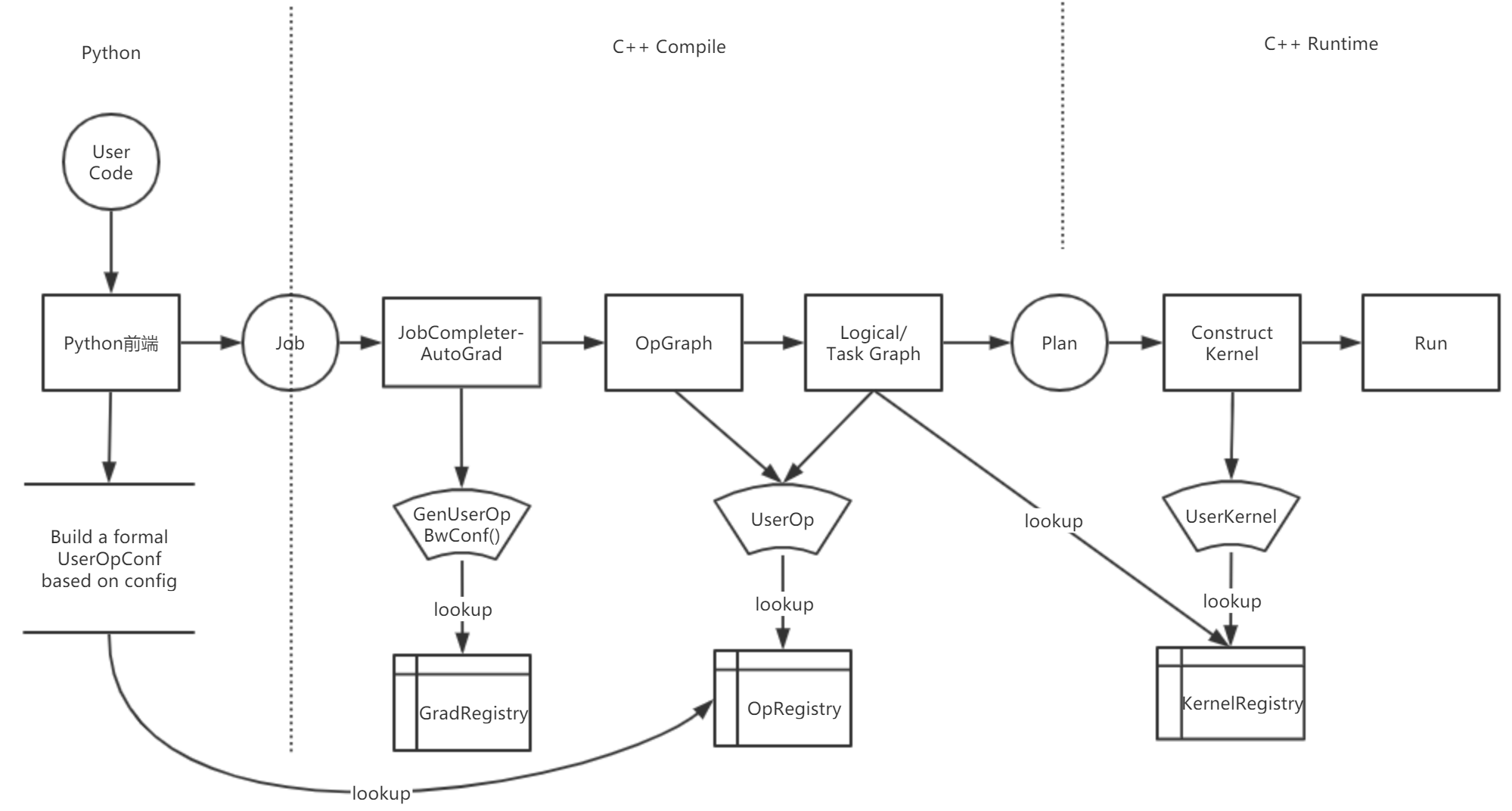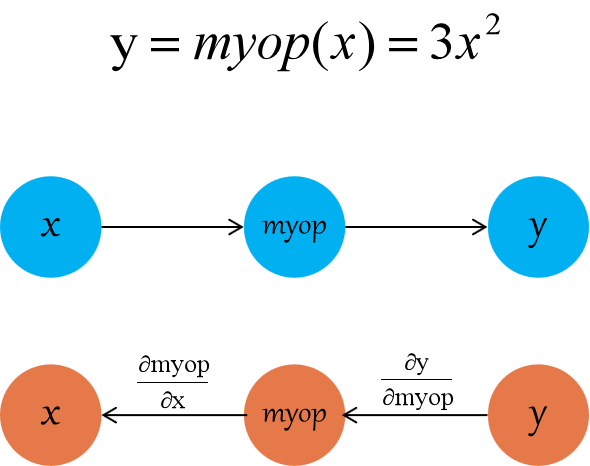# 使用 C++ 扩展 Op¶

• OneFlow 中，逻辑上的运算单元 Op 的概念
• OneFlow 中，实际负责运算的 Kernel 的概念
• Op 种类的标识符 `op_type_name` 的概念

### OneFlow 中的 Op 系统¶

OneFlow 提供了一套机制，我们在这套机制下编写自定义 op 并将其注册到 OneFlow 中，就可以在 Python 中使用自定义 op。• `OpGradRegistry`：管理梯度注册，用于反向图中自动求梯度

• `OpRegistry`：管理 op 注册，用于生成前向图及构建 `Task Graph`

• `OpKernelRegistry`：管理 kernel 注册，用于运行时执行用户编写的 kernel 逻辑

user_op_conf.proto 中可以查看 user op 的数据结构：

``````syntax = "proto2";
package oneflow;

import "oneflow/core/framework/user_op_attr.proto";

message UserOpConf {
message ListString {
repeated string s = 1;
}
required string op_type_name = 1;
map<string, ListString> input = 2;
map<string, ListString> output = 3;
map<string, UserOpAttrVal> attr = 4;
}
``````

### 使用 C++ 扩展 op 的步骤¶

1. 实现 op 并注册：op 的实现主要用于前向图构图，包括指定 op 的名称、输入、输出、配置属性以及一些必要的用于推导 tensor 的形状与数据类型的函数

2. 实现 op 对应的 kernel 并注册：kernel 负责运行时的具体运算过程，一个 op 可能会对应多个 kernel

3. （可选）实现 op 对应的 grad 并注册：如果自定义 op 需要支持后向展开，需要实现一个后向函数并注册

4. 编译链接得到 so 文件

5. 在 Python 中加载 so 文件，并且使用 `oneflow.user_op_builder` 封装 C++ 编写的自定义 op

6. 测试

## 示例¶

### op 的实现与注册¶

``````#include "oneflow/core/framework/framework.h"

namespace oneflow {

namespace {

REGISTER_USER_OP("myrelu")
.Input("in")
.Output("out")
.SetTensorDescInferFn(
[](user_op::InferContext *ctx) -> Maybe<void> {
*ctx->Shape4ArgNameAndIndex("out", 0) =
*ctx->Shape4ArgNameAndIndex("in", 0);
*ctx->Dtype4ArgNameAndIndex("out", 0) =
*ctx->Dtype4ArgNameAndIndex("in", 0);
return Maybe<void>::Ok();
});
} // namespace

} // namespace oneflow
``````

### CPU kernel 的实现与注册¶

``````#include "oneflow/core/framework/framework.h"

namespace oneflow {

namespace {

template <typename T>
void MyRelu(DeviceCtx *ctx, const int64_t n, const T *x, T *y) {
T zero = (T)(0);
for (int64_t i = 0; i != n; ++i) {
y[i] = std::max(x[i], zero);
}
}

template <DeviceType device_type, typename T>
class ReluKernel final : public user_op::OpKernel {
public:
ReluKernel() = default;
~ReluKernel() = default;

private:
void Compute(user_op::KernelComputeContext *ctx) const override {
const user_op::Tensor *in_tensor = ctx->Tensor4ArgNameAndIndex("in", 0);
user_op::Tensor *out_tensor = ctx->Tensor4ArgNameAndIndex("out", 0);
MyRelu<T>(ctx->device_ctx(),
in_tensor->shape().elem_cnt(),
in_tensor->dptr<T>(),
out_tensor->mut_dptr<T>());
}
bool AlwaysComputeWhenAllOutputsEmpty() const override { return false; }
};

#define REGISTER_RELU_KERNEL(device, dtype)          \
REGISTER_USER_KERNEL("myrelu")                     \
.SetCreateFn<ReluKernel<device, dtype>>()      \
.SetIsMatchedHob(                              \
(user_op::HobDeviceTag() == device) &     \
(user_op::HobDataType("out", 0)            \
== GetDataType<dtype>::value));

REGISTER_RELU_KERNEL(DeviceType::kCPU, float)
REGISTER_RELU_KERNEL(DeviceType::kCPU, double)
} // namespace

} // namespace oneflow
``````

• `Compute` 必须重写，在其中实现具体的运算逻辑

• `AlwaysComputeWhenAllOutputsEmpty` 必须重写，对于绝大多数 op 而言直接返回 `false` 即可。对于极少数内部需要维护状态，即使输出为空也需要调用 kernel 进行计算的 op 而言，应该返回 `true`

`REGISTER_USER_KERNEL("myrelu")` 会返回一个 `OpKernelRegistry` 对象，需要调用它的各个方法，设置注册信息。上文代码中涉及到

• `SetCreateFn<T>()`：该模板方法的模板参数 `T`，就是我们实现的 kernel 类，OneFlow 将使用它创建 kernel 对象。

• `SetIsMatchedHob`：因为一个 op 可能有多个 kernel，要想根据物理设备及数据格式的不同而选择不同的 kernel 进行计算，就需要调用 `SetIsMatchedHob` 进行设置。该方法接受一个表达式，表达式为 `true` 时，OneFlow 将调用该 kernel 完成计算。

### GPU kernel 的实现与注册¶

``````#include "oneflow/core/framework/framework.h"
#include <cub/cub.cuh>

namespace oneflow {
namespace {
template <typename T>
__global__ void ReluForwardGpu(const int n, const T *x, T *y) {
CUDA_1D_KERNEL_LOOP(i, n) { y[i] = x[i] > 0 ? x[i] : 0; }
}

class ReluGpuFloatKernel final : public user_op::OpKernel {
public:
ReluGpuFloatKernel() = default;
~ReluGpuFloatKernel() = default;

private:
void Compute(user_op::KernelComputeContext *ctx) const override {
const user_op::Tensor *in_tensor = ctx->Tensor4ArgNameAndIndex("in", 0);
user_op::Tensor *out_tensor = ctx->Tensor4ArgNameAndIndex("out", 0);

int32_t n = in_tensor->shape().elem_cnt();
const float *in_ptr = in_tensor->dptr<float>();
float *out_ptr = out_tensor->mut_dptr<float>();
ReluForwardGpu<float>
<<<32, 1024, 0, ctx->device_ctx()->cuda_stream()>>>(n, in_ptr, out_ptr);
}

bool AlwaysComputeWhenAllOutputsEmpty() const override { return false; }
};

#define REGISTER_RELU_KERNEL(device, dtype)          \
REGISTER_USER_KERNEL("myrelu")                     \
.SetCreateFn<ReluGpuFloatKernel>()             \
.SetIsMatchedHob(                              \
(user_op::HobDeviceTag() == device) &     \
(user_op::HobDataType("out", 0)            \
== GetDataType<dtype>::value));

REGISTER_RELU_KERNEL(DeviceType::kGPU, float)
REGISTER_RELU_KERNEL(DeviceType::kGPU, double)
} // namespace
} // namespace oneflow
``````

• 因为使用了 CUDA 编程，所以包含了 CUDA 对应的头文件

• `Compute` 内部使用了 GPU 的方法

• `SetIsMatchedHob` 中所匹配的设备为 GPU

### 编译链接选项说明¶

`oneflow.sysconfig` 下包含了 `get_compile_flags``get_include``get_lib``get_link_flags` 方法分别对应自定义 op 时的：

• 编译选项
• 头文件路径
• 链接库路径
• 链接选项

``````>>> import oneflow
>>> oneflow.sysconfig.get_compile_flags()
['-I/home/yaochi/oneflow/build/python_scripts/oneflow/include', '-DHALF_ENABLE_CPP11_USER_LITERALS=0', '-DWITH_CUDA', '-D_GLIBCXX_USE_CXX11_ABI=0']
``````

``````python -c "import oneflow; print(' '.join(oneflow.sysconfig.get_compile_flags()))"
python -c "import oneflow; print(' '.join(oneflow.sysconfig.get_link_flags()))"
``````

### 编译、链接得到动态库¶

``````CFLAGS = \$(shell python -c "import oneflow; print(' '.join(oneflow.sysconfig.get_compile_flags()))")
LFLAGS = \$(shell python -c "import oneflow; print(' '.join(oneflow.sysconfig.get_link_flags()))")
CUDAPATH = /usr/local/cuda-10.1/lib64

all: final_relu.so

myrelu_op.o: myrelu_op.cpp
g++ -std=c++11 -c myrelu_op.cpp \
-o myrelu_op.o                  \
-fPIC                           \
\${CFLAGS}                       \
\${LFLAGS}                       \
-O2

myrelu_cpu_kernel.o: myrelu_cpu_kernel.cpp
g++ -std=c++11 -c myrelu_cpu_kernel.cpp \
-o myrelu_cpu_kernel.o                  \
\$(CFLAGS) -fPIC

myrelu_gpu_kernel.o: myrelu_gpu_kernel.cu
nvcc -std=c++11 -c myrelu_gpu_kernel.cu \
-o myrelu_gpu_kernel.o                  \
\$(CFLAGS) -x cu -Xcompiler -fPIC

final_relu.so: myrelu_op.o myrelu_cpu_kernel.o myrelu_gpu_kernel.o
g++ -std=c++11 myrelu_op.o \
myrelu_cpu_kernel.o        \
myrelu_gpu_kernel.o        \
-shared -o final_relu.so   \
\$(CFLAGS)                  \
-fPIC                      \
-L\$(CUDAPATH)              \
-lcudart                   \
\$(LFLAGS)

clean:
rm -rf *.so *.o
``````

### 在 Python 使用自定义 op¶

• 使用 `oneflow.config.load_library` 加载 so 文件

• 使用 `oneflow.user_op_builder` 生成自定义 op 的 Python wrapper

• 调用以上的 Python wrapper 得到结果

``````import oneflow as flow
import numpy as np
import oneflow.typing as tp

# 加载模块

# 默认配置
flow.config.gpu_device_num(1)

# python op wrapper function
def myrelu(input_blob):
op = (
flow.user_op_builder("op_myrelu")
.Op("myrelu")
.Input("in", [input_blob])
.Output("out")
.Build()
)
return op.InferAndTryRun().SoleOutputBlob()

# 网络代码
@flow.global_function()
def MyJob(x: tp.Numpy.Placeholder((5,), dtype=flow.float32)) -> tp.Numpy:
return myrelu(x)

if __name__ == "__main__":
input = np.array([-2, -1, 0, 1, 2], dtype=np.float32)
output = MyJob(input)
print(input)
print(output)
``````

``````[-2. -1.  0.  1.  2.]
[0. 0. 0. 1. 2.]
``````

`myrelu` 内部构建 python wrapper 与《使用 Python 扩展 Op 》中的封装 Op 的 Python 接口代码功能完全一样，在此不再重复解释。

## OpRegistry 详细介绍¶

### `Attr` 方法¶

``````OpRegistry& Attr<cpp_type>(const std::string& name);
``````

``````REGISTER_USER_OP("reshape")
.Input("in")
.Output("out")
.Attr<shape>("shape")
``````
``````REGISTER_USER_OP("conv2d")
.Input("in")
.Input("weight")
.Output("out")
``````

OneFlow 目前支持了如下几种 C++ 数据类型：

UserOpAttrType 对应的C++数据类型
kAtInt32 int32_t
kAtInt64 int64_t
kAtBool bool
kAtFloat float
kAtDouble double
kAtShape oneflow::Shape
kAtListInt32 std::vector
kAtListInt64 std::vector
kAtListFloat std::vector< float >
kAtString std::string

``````.Attr<bool>("is_transpose", false)

.Attr<int32_t>("size", 10)

.Attr<std::vector<int32_t>>("vector_of_size", std::vector<int32_t>{10, 11, 12})
``````

### `SetCheckAttrFn` 方法¶

``````.Attr<std::string>("data_format", "NCHW")
.SetCheckAttrFn(
[](const user_op::UserOpDefWrapper& def,
const user_op::UserOpConfWrapper& conf) -> Maybe<void> {
std::string data_format = conf.attr<std::string>("data_format");
if (data_format == "channels_first" || data_format == "channels_last") {
return Maybe<void>::Ok();
}
return oneflow::Error::CheckFailed()
<< "data_format value: "
<< data_format
<< " for Conv op is illegal.";
})
``````

### 多输入/输出¶

``````// input 必须对应有1个 blob
.Input("input")

// input 必须对应有5个 blob
.Input("input", 5)

// input 必须对应至少5个 blob
.InputWithMinimum("input", 5)

// input 可能没有对应的 blob，若有则须对应1个
.OptionalInput("input")

// input 可能没有对应的 blob，若有则须对应5个
.OptionalInput("input", 5)

// input 可能没有对应的 blob，若有则须对应至少5个
.OptionalInputWithMininum("input", 5)
``````

### SetGetSbpFn 方法¶

`SetGetSbpFn` 用于设置该 `op`SBP。 以 "add_n" op 为例：

``````REGISTER_USER_OP("add_n")
.InputWithMinimum("in", 2)
.Output("out")
.SetGetSbpFn([](user_op::SbpContext* ctx) {
int64_t num_axes = ctx->LogicalTensorDesc4InputArgNameAndIndex("in", 0).shape().NumAxes();
for (int64_t i = 0; i < num_axes; ++i) {
ctx->NewBuilder().Split(ctx->inputs(), i).Split(user_op::OpArg("out", 0), i).Build();
}
ctx->NewBuilder().PartialSum(ctx->inputs()).PartialSum(user_op::OpArg("out", 0)).Build();
return Maybe<void>::Ok();
});
``````

## OpKernelRegistry 详细介绍¶

### SetInferTmpSizeFn 方法¶

``````REGISTER_USER_KERNEL("XOp")
.SetInferTmpSizeFn(
[](const oneflow::user_op::InferContext*) {
return 1024;
});
``````

``````class XKernel final : public oneflow::user_op::OpKernel {
void Compute(oneflow::user_op::KernelComputeContext* ctx) override {
oneflow::user_op::Tensor* tmp = ctx->Tensor4ArgNameAndIndex("tmp_buffer", 0);

//转换后得到 1024 字节的 char* 缓冲区
char* pBuff = tmp->mut_dptr<char>();

...
}
};
``````

Oneflow 在后向计算图展开过程中会自动求导，OneFlow 框架采用 Automatic Differentiation 方法求导，即利用链式法则自动求出整个表达式的梯度。

1. 使用 `ctx->DefineOp()``BackwardOpBuilder` 来表示计算输入的梯度的方法，因为输入的梯度计算可能是多种运算的组合，因此 `DefineOp``BackwardOpBuilder` 可能被多次使用；

2. 经过上一步定义了计算过程后，最终在某个算子的输出中，记录了需要的梯度。我们需要调用 `ctx->FwOp().InputGradBind()` 方法，将上一步的计算结果和自定义 op 的输入梯度绑定。`myop` 的前向 op 定义如下：

``````REGISTER_USER_OP("myop").Input("in").Output("out").SetTensorDescInferFn(
[](user_op::InferContext *ctx) -> Maybe<void> {
*ctx->Shape4ArgNameAndIndex("out", 0) =
*ctx->Shape4ArgNameAndIndex("in", 0);
*ctx->Dtype4ArgNameAndIndex("out", 0) =
*ctx->Dtype4ArgNameAndIndex("in", 0);
return Maybe<void>::Ok();
});
``````
`myop` 包含唯一的输入 `in` 和唯一的输出 `out`

`myop` 的反向梯度注册代码如下：

``````REGISTER_USER_OP_GRAD("myop").SetBackwardOpConfGenFn(
[](user_op::BackwardOpConfContext* ctx) {

const auto op1_name = ctx->FwOp().op_name() + "_grad1";

// 算子 op1_name 用于计算 myop.in*(myop.out的梯度)
ctx->DefineOp(op1_name,
[&ctx](user_op::BackwardOpBuilder& builder) {
return builder.OpTypeName("multiply")
.InputBind("x", ctx->FwOp().input("in", 0)) //multiply.x <- myop.in
.InputBind("y", ctx->FwOp().output_grad("out", 0)) //multiply.y <- myop.out的梯度
.Output("out")
.Build();
});

const auto op2_name = ctx->FwOp().op_name() + "_grad2";
// 算子 op2_name 用于计算 6*op1_name
ctx->DefineOp(op2_name,
[&ctx, &op1_name](user_op::BackwardOpBuilder& builder) {
return builder.OpTypeName("scalar_mul")
.InputBind("in", ctx->GetOp(op1_name).output("out", 0))
.Attr("has_float_operand", true)
.Attr("has_int_operand", false)
.Attr("float_operand", static_cast<double>(6))
.Attr("int_operand", static_cast<int64_t>(6))
.Output("out")
.Build();
});

// (myop.in的梯度) <- op1_name.out
[&ctx, &op2_name]() -> const std::string& {
return ctx->GetOp(op2_name)
.output("out", 0);
});
});
``````

`REGISTER_USER_OP_GRAD("myop")` 接受的字符串参数是 `op_type_name`，需要与 `REGISTER_USER_OP` 注册时的一致。

`REGISTER_USER_OP_GRAD("myop")` 会返回一个 `oneflow::user_op::OpGradRegistry` 对象，我们通过调用它的方法，设置自定义 op 的后向生成函数。

``````// 算子 op1_name 用于计算 myop.in*(myop.out的梯度)
ctx->DefineOp(op1_name,
[&ctx](user_op::BackwardOpBuilder& builder) {
return builder.OpTypeName("multiply")
.InputBind("x", ctx->FwOp().input("in", 0)) //multiply.x <- myop.in
.InputBind("y", ctx->FwOp().output_grad("out", 0)) //multiply.y <- myop.out的梯度
.Output("out")
.Build();
});
``````

``````// 算子 op2_name 用于计算 6*op1_name
ctx->DefineOp(op2_name,
[&ctx, &op1_name](user_op::BackwardOpBuilder& builder) {
return builder.OpTypeName("scalar_mul")
.InputBind("in", ctx->GetOp(op1_name).output("out", 0))
.Attr("has_float_operand", true)
.Attr("has_int_operand", false)
.Attr("float_operand", static_cast<double>(6))
.Attr("int_operand", static_cast<int64_t>(6))
.Output("out")
.Build();
});
``````

``````// (myop.in的梯度) <- op1_name.out
[&ctx, &op2_name]() -> const std::string& {
return ctx->GetOp(op2_name)
.output("out", 0);
});
``````

### SetBackwardOpConfGenFn 方法¶

``````void fn(BackwardOpConfContext* ctx);
``````

`BackwardOpConfContext* ctx` 带有生成 op 所需要的信息。

### BackwardOpConfContext 详细介绍¶

`BackwardOpConfContext` 类中的常用方法及其作用如下：

• `UserOpWrapper& FwOp();`：获取前向 op

• `GetOp(op_name)`: 根据 `op_name` 创建并获取对应的 `op``GetOp` 采用延迟创建机制(lazy init)，只有 `GetOp` 被调用时，对应的 op 才会被真正创建

• `void DefineOp(op_name, fn)`：定义名为 `op_name` 的 Op 的创建函数 `fn`。当调用 `ctx->GetOp(op_name)` 时， 在 OneFlow 框架中会触发 `fn` 进行 Op 创建，如果 Op 已经被创建过，那么这里直接获取创建的结果。`fn` 函数接收一个 `BackwardOpBuilder` 参数，用于构建反向 op，我们接下来介绍 `BackwardOpBuilder`

### BackwardOpBuilder 详细介绍¶

`BackwardOpBuilder` 用于构建一个反向 op。以上文中的代码片段为例

``````ctx->DefineOp(op1_name,
[&ctx](user_op::BackwardOpBuilder& builder) {
return builder.OpTypeName("multiply")
.InputBind("x", ctx->FwOp().input("in", 0)) //multiply.x <- myop.in
.InputBind("y", ctx->FwOp().output_grad("out", 0)) //multiply.y <- myop.out的梯度
.Output("out")
.Build();
});
``````

• `OpTypeName("multiply")` 指定一个 op 的 `op_type_name`，使用这个 op 来帮助我们进行反向梯度的计算

• `InputBind(arg_name, blob)``multiply` 的输入 `arg_name` 与 指定的 `blob` 进行绑定，可以调用多次，如果该 `arg_name` 对应多个输入blob，则调用 `Input` 的顺序就是其对应的 index 顺序

• `Output(arg_name, num)` 指定一个 `arg_name` 实际对应的输出 blob 的数量，如果不填 `num`，则 `num` 默认为1

• `Attr(attr_name, val)` op 设置属性值，与注册 op 时的用法一样

• `Build()` 完成各种设置后，通过调用 `Build` 完成反向 op 的构建

### UserOpWrapper 详细介绍¶

``````ctx->FwOp().InputGradBind(user_op::OpArg("in", 0),
[&ctx, &op2_name]() -> const std::string& {
return ctx->GetOp(op2_name)
.output("out", 0);
});
``````

`UserOpWrapper` 的常见方法有：

• `InputGradBind(input, grad_fn)`：绑定前向 op 的输入与获取梯度的函数 `grad_fn`。 OneFlow 会自动判断 `input` 是否需要生成后向的梯度，如果需要则触发 `grad_fn` 并进行绑定；

• `input(arg_name, index)`：得到输入 `arg_name` 对应的 blob

• `output(arg_name,index)`：得到输出 `arg_name` 对应的 blob

• `output_grad(output_arg_name, index)`： 返回前向 op 的输出 `output_arg_name` 对应的后向梯度的 blob

• `attr(attr_name)`：获取属性 `attr_name` 对应的值

• `arg_tensor_desc(arg_name, index)`：返回前向 op 的输入/输出对应的 tensor 信息，包含 `shape``dtype`

## UserOpConfBuilder 详细介绍¶

``````def cast(x, dtype, name):
return (
flow.user_op_builder(name)
.Op("cast")
.Input("in", [x])
.Output("out")
.Attr("dtype", dtype)
.Build()
.InferAndTryRun()
.RemoteBlobList()
)
)
``````

• `Op(op_type_name)`：接受的参数为 C++ 中注册时的 `op_type_name`

• `Input(input_name, input_blob_list)`：输入，`input_name` 应与 C++ 中注册 op 时 `Input` 的第一个参数一致

• `Output(output_name, num=1)`：输出，`output_name``num` 应与 C++ 中注册 op 时的 `Output` 一致

• `Attr(attr_name, attr_value)`：设置属性，`attr_name` 对应了 C++ 注册时使用 `OpRegistry::Attr` 声明的属性，且 `attr_value` 类型应当与声明时的属性类型一致

• `Build()`：构建得到 Python 层的 user op

• `RemoteBlobList`：获取所有输出，适用于有多个输出的 op，所有的 op 放置在一个 list 中

• `SoleOutputBlob`：获取唯一的输出，适用于只有一个输出的 op